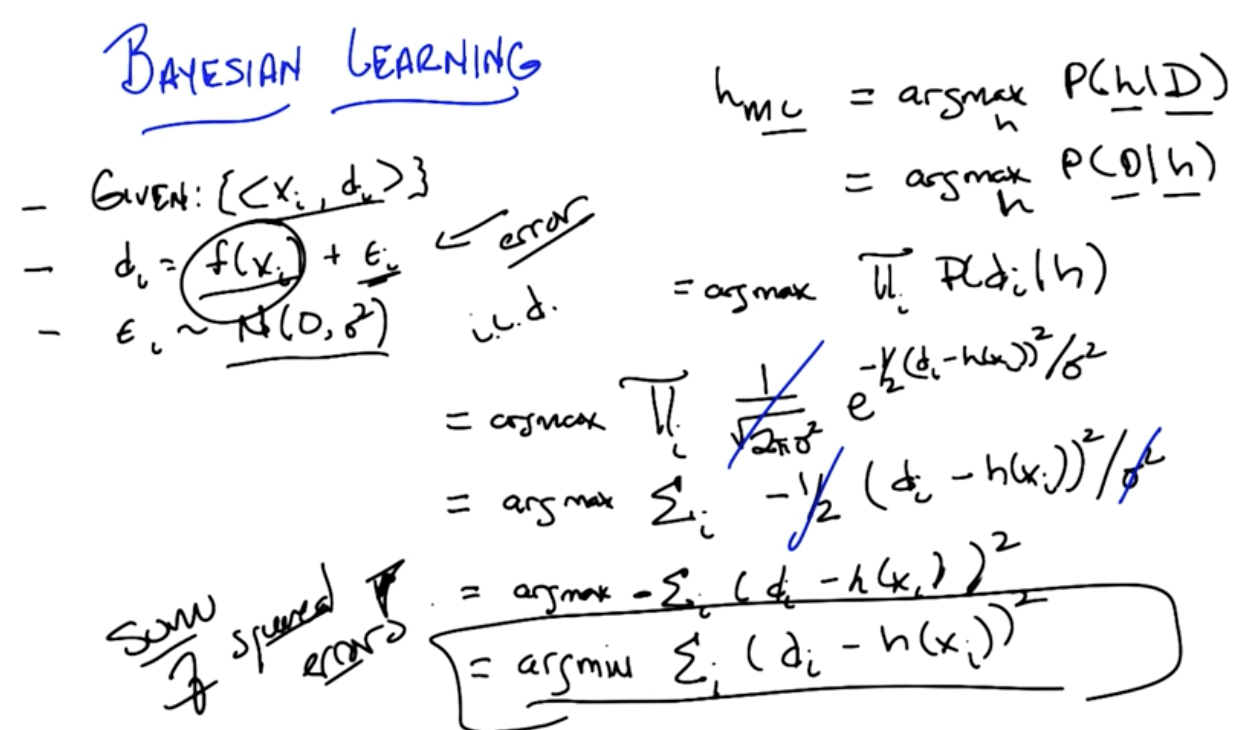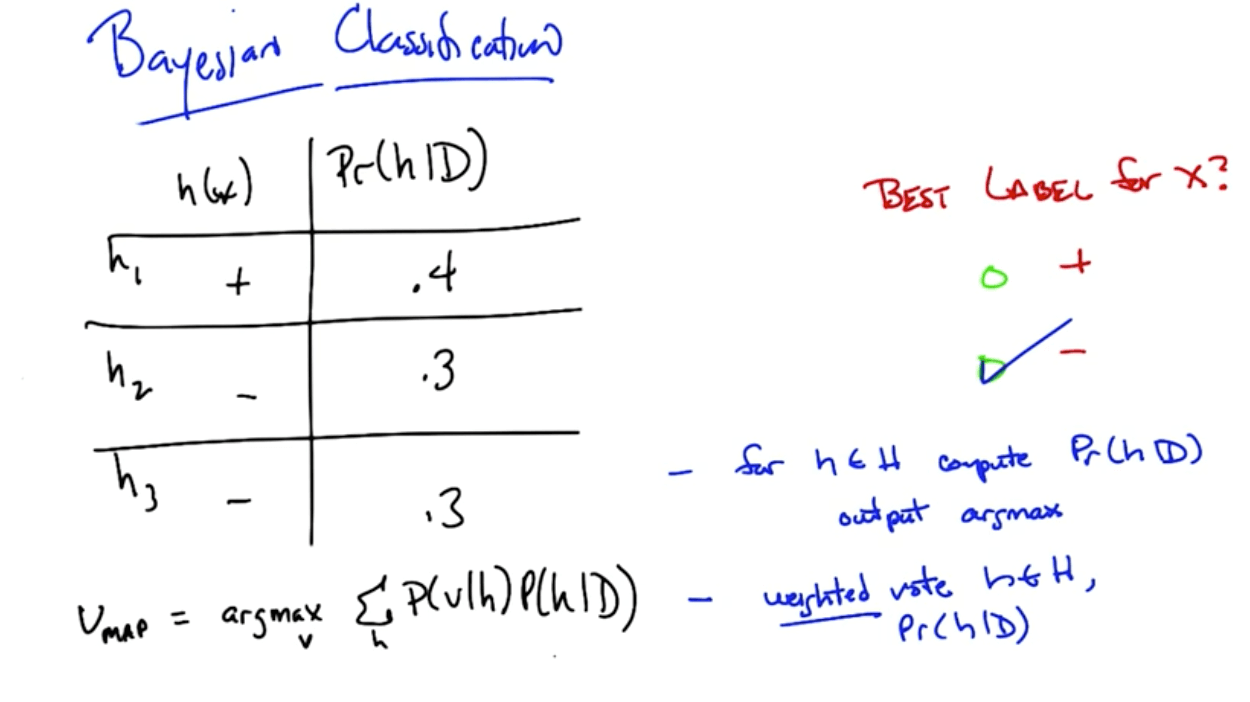Gaussian naive bayes, bayesian learning, and bayesian networks
Gaussian Naive Bayes

## Naive Bayes Methods¶

Bayes Rule: Intuitive Explanation

• (Prior probability)(Test evidence) --> (Posterior probability)
• Example
• P(C) = 0.01
• 90% it is positive if you have C (Sensitivity)
• 90% it is negative if you don't have C (Specificity)
• prior
• P(C) = 0.01
• P(C') = 0.99
• P(Pos|C) = 0.9
• P(Pos|C') = 0.1
• P(Neg|C') = 0.9
• P(Neg|C) = 0.1
• joint
• P(C and Pos) = P(C)P(Pos|C) = (0.01)(0.9) = 0.009
• P(C'and Pos) = P(C')P(Pos|C') = (0.99)(0.1) = 0.099
• normalizer
• P(Pos) = P(C and Pos) + P(C' and Pos) = 0.108
• posterior
• P(C|Pos) = 0.009 / 0.108 = 0.0833
• P(C'|Pos) = 0.099 / 0.108 = 0.9167

Bayes Rule: Example

• This is really good for text learning
• Example
• P(Chris) = 0.5
• P(Love|Chris) = 0.1
• P(Deal|Chris) = 0.8
• P(Life|Chris) = 0.1
• P(Sara) = 0.5
• P(Love|Sara) = 0.5
• P(Love|Deal) = 0.2
• P(Love|Life) = 0.3
In :
p_chris_and_love_deal = 0.1*0.8*0.5

p_sara_and_love_deal = 0.5*0.2*0.5

normalizer = p_chris_and_love_deal + p_sara_and_love_deal

p_chris_given_love_deal = p_chris_and_love_deal / normalizer
p_sara_given_love_deal = p_sara_and_love_deal / normalizer

# P(Chris | "Love Deal")
print(p_chris_given_love_deal)

# P(Sara | "Love Deal")
print(p_sara_given_love_deal)

0.4444444444444445
0.5555555555555555


Bayes Rule: Theory

• Learn the best hypothesis given data and some domain knowledge
• Learn the most probable hypothesis given data and domain knowledge
• $$\underset{h∈H}{\mathrm{argmax}}P(h|D)$$
• h: some hypothesis
• D: some data
• argmax h∈H
• We want to maximize P(h|D)
• Bayes rule
• $$P(h|D) = \frac{P(D|h)P(h)}{P(D)}$$
• $$P(a,b) = P(a|b)P(b)$$
• $$P(a,b) = P(b|a)P(a)$$
• P(a,b) is the probability of a and b
• P(D)
• This is a normalizing term
• Prior on the data
• P(D|h)
• Data given the hypothesis
• $$D =\{x_i, d_i\}$$
• Training data, D, with inputs (x) and labels (d)
• What's the likelihood that given all of x_i and P(D|h) hypothesis is true, we will observe d's
• P(h)
• Prior on h
• Domain knowledge
• Say if you use KNN, you believe points close together would give similar outputs with higher likelihood than those far from one another

Bayesian Learning Algorithm

• For each h∈H, calculate P(h|D) ≈ P(D|h)
• Output
• $$h_1 = \underset{h}{\mathrm{argmax}}P(h|D)$$
• h_1: Maximum a posteriori
• $$h_2 = \underset{h}{\mathrm{argmax}}P(D|h)$$
• h_2: Maximum likelihood
• We're assuming P(h) and P(D) are uniform
• They're constants so we can ignore

Gaussian Naive Bayes

• Ultimately we've simplified, using Gaussian distribution, to minimizing the sum of squared errors!
• Based on bayes rule we've ended up deriving sum of squared error
•Bayesian Classification

• The algorithm changes slightly here
• We are maximizing the weighted vote instead of simply P(h|D)
•Version Space

• The version space is a subset of the hypothesis space, where the hypotehesis space is a space of all possible hypotheses
• The version space are those hypotheses such that they correctly predict the training data you have (essentially a 100% model fit)

Bayesian Networks, Bayesian Nets, Belief Networks or Graphical Models

• Representing and dealing with probabilities
• Conditional independence
• X is conditionally independent of Y given Z if the probability distribution governing X is independent of the value of y given the value of Z
• P(X=x | Y=y, Z=z) = P(X=x | Z=z)
• More compactly
• P(X|Y,Z) = P(X|Z)
• Order of graph must be topological
• Graph must be acylic
• No cycles
• Sampling
• Two things distributions are for
• Probability of value
• Generate values
• Reasons for sampling
• Simulation of a complex process
• Approximate inference
• For machines
• We can't find the exact values because it may be hard and slower
• Visualization
• For humans to get a feel
• Inferencing Rules
• Marginalization
• $$P(x) = \underset{y}\sum(x,y)$$
• Chain rule
• $$P(x,y) = P(y|x)P(x) = P(y|x)P(y)$$
• Bayes rule
• $$P(y|x) = \frac {P(x|y)P(y)}{P(x)}$$

Naive Bayes

• Say you've label A and B (hidden)
• Label A
• Have multiple words with different probabilities
• Every word gives evidence if it's label A
• We mutiply all the probabilities with the prior to find the joint probability of A
• Label B
• Have multiple words with different probabilities
• Every word gives evidence if it's label B
• We multiply all the probabilities with the prior to find the joint probability of B
• Now you can find out the probability of it being A or B
• Reason why it's called Naive
• It ignores word order!

Naive Bayes Benefits

• Inference is cheap
• Linear
• Few parameters
• Estimate parameters with labeled data
• Connects inference and classification
• Empirically successful

Naive Bayes Training

• In the training process of a Bayes calssification problem, the sample data does the following:
1. Estimate likelihood distributions of X for each value of Y
2. Estimate prior probability P(Y=j)

Gaussian Naive Bayes in Scikit-learn

In :
from sklearn.naive_bayes import GaussianNB
from sklearn.cross_validation import train_test_split
from sklearn.metrics import accuracy_score

In :
# Create features' DataFrame and response Series
X = iris.data
y = iris.target

X_train, X_test, y_train, y_test = train_test_split(X, y, random_state=6)

In :
# Instantiate: create object
gnb = GaussianNB()

# Fit
gnb.fit(X_train, y_train)

# Predict
y_pred = gnb.predict(X_test)

# Accuracy
acc = accuracy_score(y_test, y_pred)
acc

Out:
0.92105263157894735
Tags: